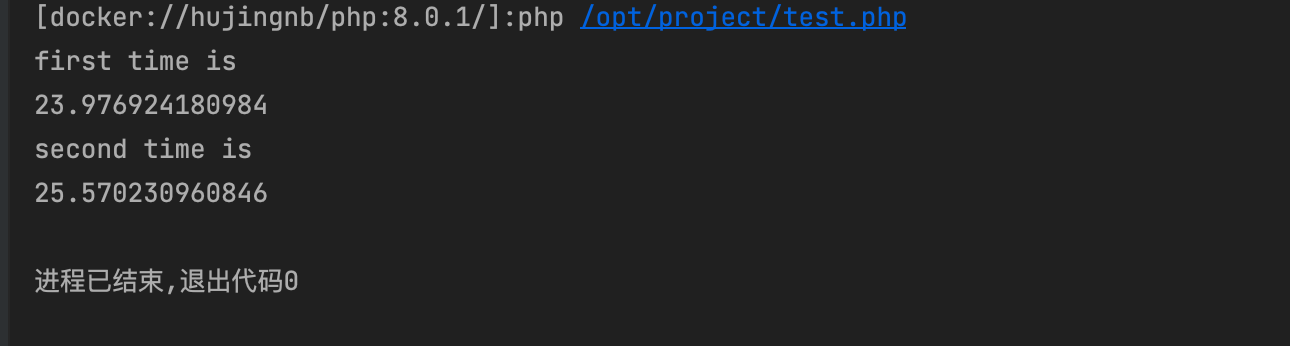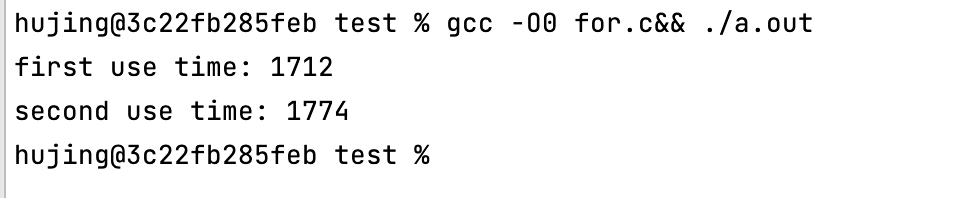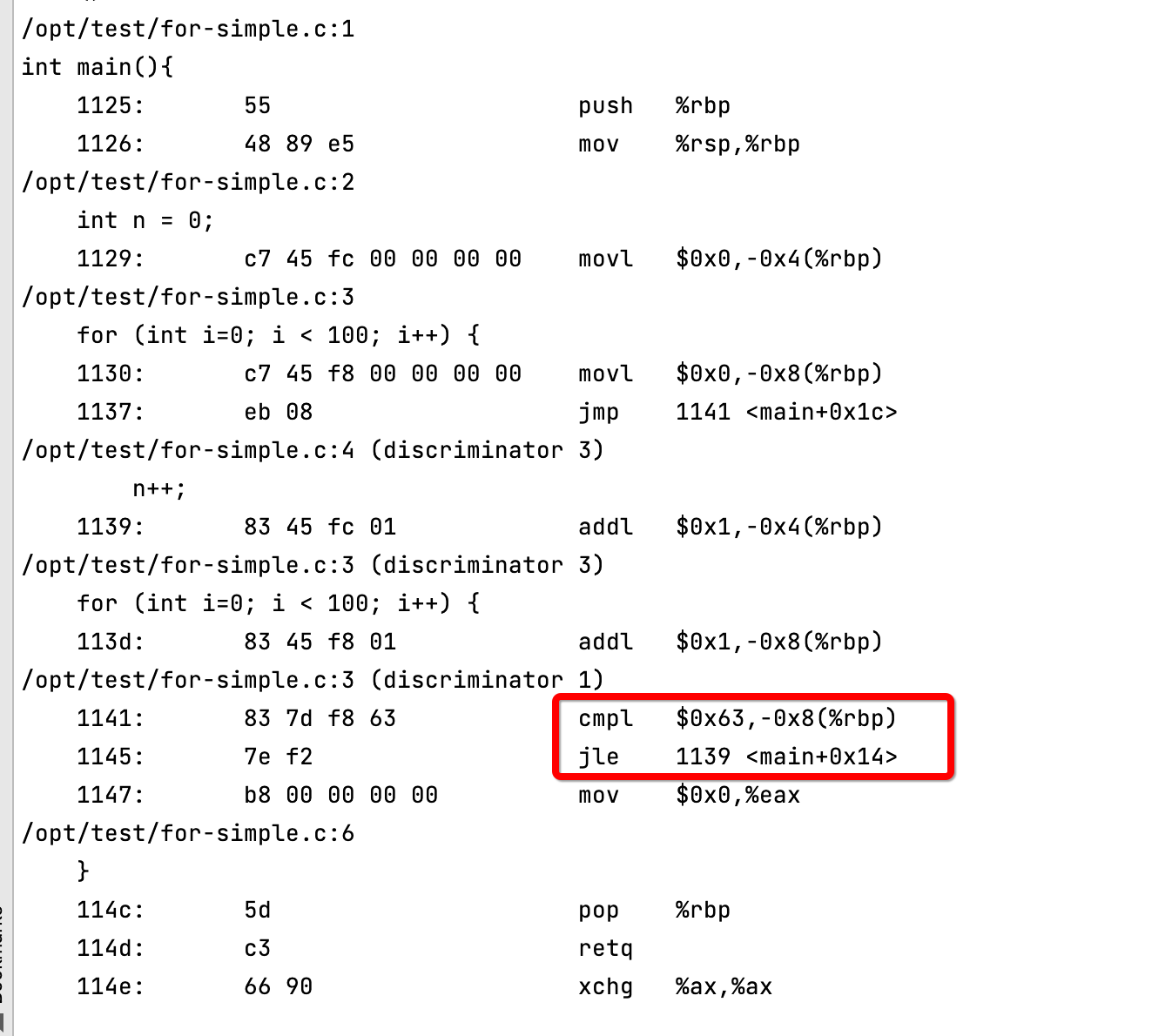# 前言

<?php
// 第一次运行
$start = microtime(true); for ($i = 0; $i < 100;$i++) {
for ($j = 0;$j <1000; $j++) { for ($k = 0;$k < 10000;$k++) {
}
}
}
$end = microtime(true); echo 'first time is ', PHP_EOL; echo$end - $start, PHP_EOL; // 第二次运行$start = microtime(true);
for ($i = 0;$i < 10000; $i++) { for ($j = 0; $j <1000;$j++) {
for ($k = 0;$k < 100; $k++) { } } }$end = microtime(true);
echo 'second time is ', PHP_EOL;
echo $end -$start, PHP_EOL;what? why? 于是我想, 有没有可能是运行顺序的原因. 因此我将第二种放到前面运行, 结果也相应的颠倒了.

# 解释

#include "stdio.h"
#include "sys/time.h"

int main(){
struct timeval t;
int n;
long startTime, endTime;
// 第一次运行
gettimeofday(&t,NULL);
startTime = t.tv_sec * 1000 + t.tv_usec / 1000;
n =  0;
for (int i=0; i < 100; i++) {
for(int j = 0; j < 1000; j++){
for (int k = 0; k < 10000; k++){
n++; // 为了防止 CPU 优化, 在循环中做了自增的操作
}
}
}
gettimeofday(&t,NULL);
endTime = t.tv_sec * 1000 + t.tv_usec / 1000;
printf("first use time: %ld\n", endTime - startTime);
// 第二次运行
gettimeofday(&t,NULL);
startTime = t.tv_sec * 1000 + t.tv_usec / 1000;
n=0;
for (int i=0; i < 10000; i++) {
for(int j = 0; j < 1000; j++){
for (int k = 0; k < 100; k++){
n++;
}
}
}
gettimeofday(&t,NULL);
endTime = t.tv_sec * 1000 + t.tv_usec / 1000;
printf("second use time: %ld\n", endTime - startTime);
}## CPU 流水线

CPU在执行指令的时候, 要经过多个步骤, 下面是一个简化的步骤:

1. 取指令
2. 指令译码
3. 执行指令
4. 输出结果## 指令预测## for 指令实现

int main(){
int n = 0;
for (int i=0; i < 100; i++) {
n++;
}
}

gcc -g for-simple.c && objdump -dSl ./a.out## 解惑

int main(){
for (int i=0; i < 100; i++) {
for(int j = 0; j < 1000; j++){
for (int k = 0; k < 10000; k++){
// CPU 在这里进行分支预测, 仅最后一次预测失败. 共失败1次
}
// CPU 在这里进行分支预测, 共失败999次, 最后一次成功
// 因为上一次第三层循环退出, 因此本次预测退出循环, 但结果继续第二层循环
}
// CPU 在这里分支预测, 共失败99次
}
}

(这里为了方便计算, 上面的99都近似为100进行计算了, 否则数字根本没法看)0 评论

0